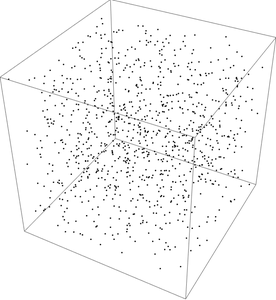# Expected distance between points in a cubeSuppose you randomly sample points from a unit cube. How far apart are pairs of points on average?

My intuition would say that the expected distance either has a simple closed form or no closed form at all. To my surprise, the result is somewhere in between: a complicated closed form.

Computing the expected value by simulation is straight forward. I’ll make the code a little less straight forward but more interesting and more compact by leveraging a couple features of NumPy.

    import numpy as np

dimension = 3
reps = 10000

cube1 = np.random.random((reps, dimension))
cube2 = np.random.random((reps, dimension))
distances = np.linalg.norm(cube1 - cube2, axis=1)
print(distances.mean())


This gives a value of 0.6629. This value is probably correct to two decimal places since the Central Limit Theorem says our error should be on the order of one over the square root of the number of reps.

In fact, the expected value given here iswhich evaluates numerically to 0.661707….

The expression for the expected value is simpler in lower dimensions; it’s just 1/3 in one dimension. In higher dimensions there is no closed form in terms of elementary functions and integers.

Incidentally, the image above was made using the following Mathematica code.

    pts = RandomPoint[Cube[], 10^3];
Graphics3D[{PointSize[Small], Point[pts], Opacity[0.3]}, Boxed -> True]


## 3 thoughts on “Expected distance between points in a cube”

1. Srinjoy Majumdar

Since the points are distributed randomly, I considered the distance each pair to be distributed randomly as well. So, let X be the rv associated with the distance between a pair of points and since √3 is the max distance possible, X~U[0,√3].
Thus the expected distance turns out to be √3/2.
Can you please explain where I have gone wrong?

2. You are correct that the distances are between 0 and √3, but it is incorrect to assume they are uniformly distributed.

In dimension n, the distances are between 0 and √n. To make things easy, let n = 1. If the distances between points in the unit interval were uniformly distributed, the mean would be 1/2. But the actual mean is 1/3.

You can tell that 1/2 is too high because it’s the average distance to one end of the interval, say 0. But typically the leftmost point is not as far to the left as possible.